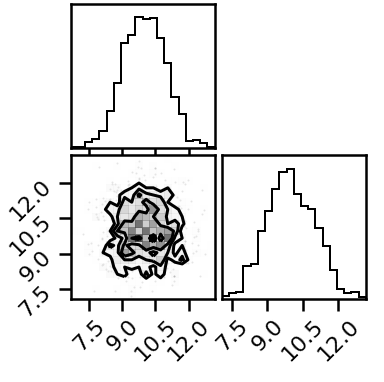# Drawing samples from a Gaussian Mixture

Hi all!

I’m trying to draw samples from a MixtureSameFamily of MultivariateNormal distributions. However when I sample from the model below I only seem to sample from one of the MVNormal distributions, and ignoring the other. Does anybody know what I"m doing wrong here? I’m using Numpyro in Python.

Cheers!
Oliver

``````def model():
mixing_dist = dist.Categorical(probs = jnp.array([0.3, 0.7]))
locs = jnp.array([[1, 1], [10, 10]])
covs = jnp.array([[[1, 0],
[0, 1]],
[[1, 0],
[0, 1]]])

component_dist = dist.MultivariateNormal(
loc = locs,
covariance_matrix = covs)

npy.sample('y', dist.MixtureSameFamily(mixing_dist, component_dist))

nuts = NUTS(model, init_strategy=npy.infer.init_to_median)
mcmc = MCMC(nuts, num_warmup = 0, num_samples=1000)

rng, key = random.split(rng)
mcmc.run(key)

corner(mcmc.get_samples()['y']);
``````HMC/NUTS is not good at dealing with multi-modal posteriors. To remedy the issue, one way is to follow the approach in Neural Transport tutorial.

Hey @fehiepsi! In this case I just want to draw samples from a multi-modal distribution for which I know all the details (means and covariance matrix). I can get this working fine in a 1-component case, its just the generalising to multiple components that I’m struggling to get right.

Yes, NUTS gets trouble with multi-modal distributions. (By posterior, I meant the target distribution that we want to sample from - sorry for confusion).

Hi @fehlespi!

I’ve been hacking away at this today with no luck, but I’ve figured some things out. Both the vanilla HMC sampler and the NeuTra HMC sampler (as used in that tutorial) seem to both struggle with sampling bimodal distributions. Perhaps this is a problem with initial guesses. See code below.

I was wondering whether a Nested Sampler would be the way to go instead, as I hear they’re better at drawing from multimodal distributions. However following this example (Example: Nested Sampling for Gaussian Shells — NumPyro documentation) results in a `NotImplementedError` for me, so wasn’t sure if the method was fully functional yet in Numpyro.

``````class mixmodel_2D(dist.Distribution):
support = constraints.real_vector

def __init__(self):
super(mixmodel_2D, self).__init__(event_shape=(2,))

def sample(self, key, sample_shape=()):
return jnp.zeros(
sample_shape + self.shape()
)  # a dummy sample to initialize the samplers

# Multivariate normal distribution, takes means and covariance
def gauss_mv(self, x, locs, covs):
d = len(locs)
xm = x - locs
A = (jnp.dot(jnp.dot(xm, jnp.linalg.inv(covs)), xm.T))
B = d*jnp.log(2*jnp.pi) + jnp.log(jnp.linalg.det(covs))
return -0.5 * (A+B)

def log_prob(self, x):
locs = np.array([[-15, 10],
[-15, 20],
[-5, 15]])
cov = np.array([[5, 0],
[0, 5]])
covs = jnp.array([cov, cov, cov])
weights = jnp.array([0.1, 0.3, 0.6])

terms = jnp.array([self.gauss_mv(x, locs[i], covs[i]) for i in range(len(locs))])

args = jnp.array([terms[i] + jnp.log(weights[i]) for i in range(len(locs))])

ll = jnp.sum(jnp.log(jnp.sum(jnp.array([jnp.exp(args[i]) for i in range(len(locs))]),axis=0)))
return ll

def model():
npy.sample('y', mixmodel_2D())

num_warmup = 100
num_samples = 1000
num_chains = 1
num_iters = 1000

## First a regular NUTS HMC
nuts_kernel = NUTS(model)
mcmc = MCMC(
nuts_kernel,
num_warmup=num_warmup,
num_samples=num_samples,
num_chains=num_chains,
)
mcmc.run(key)
mcmc.print_summary()
vanilla_samples = mcmc.get_samples()["y"].copy()

#Now the NeuTra HMC
guide = AutoBNAFNormal(
model, hidden_factors=[8, 8]
)
svi = SVI(model, guide, optim.Adam(0.003), Trace_ELBO())

print("Start training guide...")
svi_result = svi.run(key, num_iters)
print("Finish training guide. Extract samples...")
guide_samples = guide.sample_posterior(
random.PRNGKey(2), svi_result.params, sample_shape=(num_samples,)
)["y"].copy()

print("\nStart NeuTra HMC...")
neutra = NeuTraReparam(guide, svi_result.params)
neutra_model = neutra.reparam(model)
nuts_kernel = NUTS(neutra_model)
mcmc = MCMC(
nuts_kernel,
num_warmup=num_warmup,
num_samples=num_samples,
num_chains=num_chains,
)
mcmc.run(random.PRNGKey(3))
mcmc.print_summary()
zs = mcmc.get_samples(group_by_chain=True)["auto_shared_latent"]
print("Transform samples into unwarped space...")
samples = neutra.transform_sample(zs)
print_summary(samples)
zs = zs.reshape(-1, 2)
samples = samples["y"].reshape(-1, 2).copy()
``````

In the figures below, the red dots indicate the locations of the `locs` for the three component gaussians in the distribution - the NeuTraHMC sampler doesn’t touch them at all.